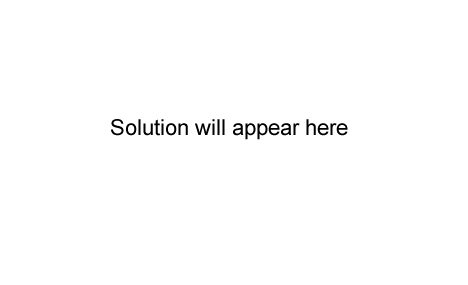Gravimetric exercises (2006) Question 1 The concentration of K+ ions in 100 mL of 0.0500 M K2CO3 solution, in g L-1, is A. 0.196 B. 0.391 C. 1.96 D. 3.91 Click here for solutionQuestion 2 Carbon monoxide can be oxidised to carbon dioxide. 2CO(g) + O2(g) → 2CO2(g) 3 mol of CO and 2 mol of O2 are mixed. When the reaction is complete there will be A. 4 mol of CO2 produced. B. 2 mol of CO2 produced. C. 1 mol of CO unreacted. D. 0.5 mol of O2 unreacted. Click here for solutionA soluble fertiliser contains phosphorus in the form of phosphate ions (PO43-). To determine the PO43- content by gravimetric analysis, 5.97 g of the fertiliser powder was completely dissolved in water to make a volume of 250.0 mL. A 20.00 mL volume of this solution was pipetted into a conical flask and the PO43- ions in the solution were precipitated as MgNH4PO4. The precipitate was filtered, washed with water and then converted by heating into Mg2P2O7. The mass of Mg2P2O7 was 0.0352 g. a. Calculate the amount, in mole, of Mg2P2O7.   Click here for solutionb. Calculate the amount, in mole, of phosphorus in the 20.00 mL volume of solution. Click here for solutionc. Calculate the amount, in mole, of phosphorus in 5.97 g of fertiliser. Click here for solutiond. Calculate the percentage of phosphate ions (PO43-) by mass in the fertiliser. Ensure you express your answer to an appropriate number of significant cant figures. Click here for solutionContinue with more gravimetric analysis exercises from past exam papers